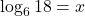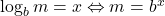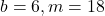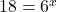## Which exponential equation correctly rewrites this logarithmic equation? log6 18=x

Question

Which exponential equation correctly rewrites this logarithmic equation? log6 18=x

in progress 0
4 months 2021-09-05T12:08:00+00:00 1 Answers 3 views 0

## Answers ( )

1. Given:

The logarithmic equation is:To find:

The exponential equation of this logarithmic equation.

Solution:

According to the properties of logarithm:We have,Here,. Using the above property of logarithm, we getTherefore, the required exponential equation is.